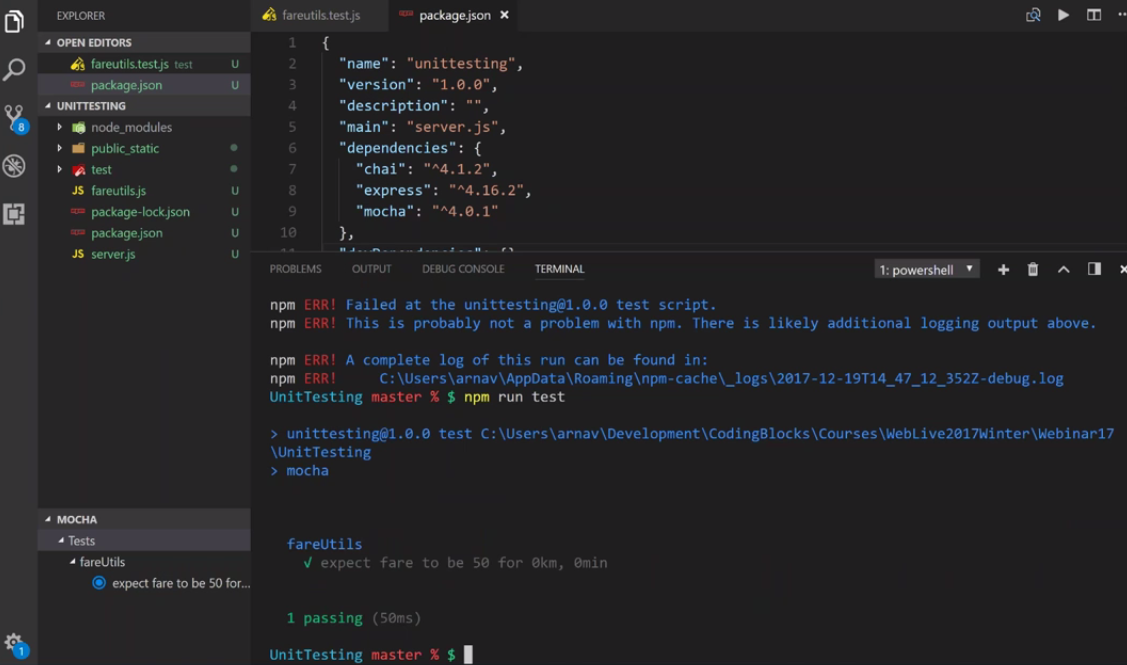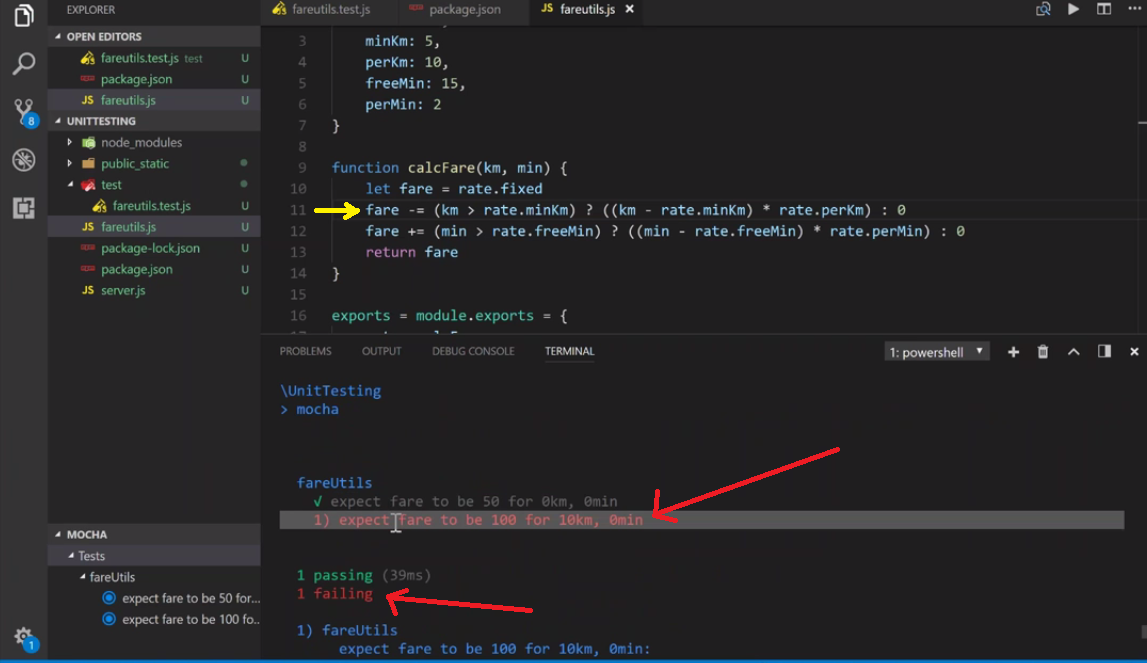Related Articles

# Debugging and Testing of a Node Application

• Difficulty Level : Expert
• Last Updated : 24 Jul, 2019

While building a big project working in a team, continuous changes being in code base are going to achieve optimum results. So if someone writes code and because of which an already existing feature gets broken, for that purpose we use software testing.

Keeping your project tested automatically we have tests, every time we run the project, we can check which test continue to pass and which are failing to pass. Those tests which fail to pass indicate that some changes are being made in that section of the code.

Develop a simple web app for Implementing Testing and Debugging: In this web app we have fares set according to various parameters and if someone makes changes in the fares we have to get them tested so that every member of the team gets aware that changes in fare rates have taken place.

Step 1: Create a project folder

```npm init
npm install express
```

Step 2: Add your logic to be tested in a separate file in root folder
In this file (fareutils.js) we store the code which we want to test and will import the functions to our server file.

 `// Shows the user rate chart``rate =  {``    ``fixed: 50,``    ``minKm: 5,``    ``perKm: 10,``    ``freeMin: 15,``    ``perMin: 2``}`` ` `// Logic for calculating fare according to ride details``function` `calcFare(km, min) {``    ``let fare = rate.fixed``    ``fare += (km > rate.minKm) ? ((km - rate.minKm) * rate.perKm) : 0``    ``fare += (min > rate.freeMin) ? ((min - rate.freeMin) * rate.perMin) : 0``    ``return` `fare``}`` ` `// Exports the two functions to server.js``exports = module.exports = {``    ``rate, calcFare``}`

Step 3: Now implement tests of the above logic we use MOCHA and CHAI:

• Mocha is a JS testing framework. It uses describe method to group our tests and it method to run our tests.
• Chai is an assertion library that makes sure that our values are similar because chai can equate objects and arrays which is not easy to do with plain javascript. It does a deep-equal check that checks and also equates the contents of objects.
```npm install mocha chai
```

Step 4: Create a new folder called test in the root directory:
We will make a file (fareutils.test.js) in which we will write our mocha and chai test cases.

 `// Importing mocha and chai``const mocha = require(``'mocha'``)``const chai = require(``'chai'``)`` ` `// Importing fareutils.js where our code is written``const fareUtils = require(``'../fareutils'``)`` ` `const expect = chai.expect`` ` `// Group of tests using describe``describe(``'fareUtils'``, ``function` `() {`` ` `    ``// We will describe each single test using it``    ``it(``'expect fare to be 50 for 0km, 0min'``, () => {``        ``let fare = fareUtils.calcFare(0, 0)``        ``expect(fare).to.equal(50)``    ``})`` ` `    ``it(``'expect fare to be 100 for 10km, 0min'``, () => {``        ``let fare = fareUtils.calcFare(10, 0)``        ``expect(fare).to.equal(100)``    ``})`` ` `    ``it(``'expect fare to be 56 for 2km, 18min'``, () => {``        ``let fare = fareUtils.calcFare(2, 18)``        ``expect(fare).to.equal(56)``    ``})``})`

Step 5: set up the node server

 `const express = require(``'express'``);``const path = require(``'path'``)``const fareUtils = require(``'./fareutils'``)`` ` `const app = express();`` ` `app.use(express.json())``app.use(express.urlencoded({extended: ``true``}))`` ` `app.use(``'/'``, express.static(path.join(__dirname,``                    ``'public_static'``)))`` ` `app.post(``'/calcfare'``, (req, res) => {``    ``let km = parseFloat(req.body.km)``    ``let min = parseInt(req.body.min)`` ` `    ``let fare = fareUtils.calcFare(km, min)`` ` `    ``res.send({fare: fare})``})`` ` `app.get(``'/rate'``, (req, res) => {``    ``res.send(fareUtils.rate)``})`` ` `app.listen(2222, () => console.log(``    ``'Server started on http://localhost:2222'``))`

Step 6: How to run mocha test
If all of the tests are in the Test folder and if you have correctly required mocha and chai. Here you can simply run tests by describing your package.json file as

```"scripts": {
"test": "mocha",
"start": "node app.js",
}
```

Now, we will start our test by following command in terminal or command promopt:

```npm run test
```

The result of test when everything is correct and code is unchanged:so the test is passed and a green tick is displayed on the terminal.

Now if someone make changes in the fareutils.js then lets see the terminal:
Here we have made changes in line 11 of fareutils.jsMy Personal Notes arrow_drop_up